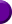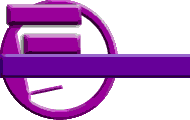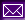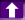The team Projects Articles FeaturesThe Z80 processor? Never heard of that one!
The Z80 is indeed a very old processor, I think it was first released in 1977 (correct me if I'm wrong). But it is still used in various hand-held calculators, and gaming consoles, as it is quite robust, fast (a 6MHz Z80 CPU is quite a bit faster than a 6MHz Intel CPU) and furthermore it doesn't need too much power.

Where is the Z80 used?
I can only state a few examples: TI-8x calculators, Nintendo Gameboy, the Sega Master System and the Sinclair Spectrum, to name a few. I believe that is also used for various simple hardware as controller.

Is the Z80 still being produced?
The Z80 is still in production, but you can't get the chip from Zilog. You have to get it from other distributers, like this one. They are really cheap, you can get the 5 MHz version for about \$2, the 20 MHz version for \$5.

Do you know any good books on programming the Z80?
"Programming the Z80" by Rodnay Zaks is BY FAR the best book on assembly language programming. There also exist some other useful ones, like "Z80 assembly language subroutines" by Lance A. Leventhal and Winthrop Saville. What's more, I suggest at least getting an opcode table, so you can look up all possible combinations while programming.
You might also find some information on the net.

An opcode - what's that?
The Z80 is able to perform various instructions. An instruction specifies the operation to be performed by the microprocessor. From a simplified standpoint, every instruction may be represented as an opcode followed by an optional literl or address field, comprising one or two words. The opcode specifies the operation to be carried out.

What is a register?
For faster access, the CPU itself contains a few "variables", so-called registers. All main operations, like math, etc. are performed using these, as they can be accessed more quickly than the main memory.
On the Z80, these registers are called: A, B, C, D, E, H, L (and IX, IY, F, I, R, SP: see below), A = accumulator being the most important one. As the Z80 is an 8-bit processor, these registers are all 8-bit wide, this means they can hold any value from 0-255 (= 8 bit or one byte). However, to store larger numbers, you can combine a few of them: BC, DE, HL. The result is a 16 bit register, having a range from 0-65535. Note that if you modify for instance B, the upper byte from BC will be modified, too: Given that BC is \$467C. Now you execute the instruction:

 ```ld B, \$58 ;load \$58 (hexadecimal) into B ```

Then B will be equal to \$58, and BC will be \$587C. Same's true for DE and HL.

You didn't mention the IX, IY, F, I, R and SP registers.
These are special purpose registers. They can be only used (or directly accessed) for few operations:

• The index registers IX and IY are mostly used as auxiliary HL. They are both 16 bit registers where you can't modify the upper/lower byte independently (without using special code). The reason for their name is that they can be used to index fields in a structure, like

 ```ld IX, \$8000 ;point IX to memory location at \$8000 ld A, (IX+\$10);load A with value of memory location ... ;at IX+\$10 = \$8010 ```

This is of course comfortable in some cases, however, it is suggested to avoid using the index registers, as the execution is slower and one opcode needs more memory.
• The F (flag) register is perhaps the most important one of those listed here. It contains the so-called flags, which store certain conditions after executing opcodes. The flag register is basically a normal 8 bit register, but each of the eight bits represent a flag:
```| S | Z |   | H |P/V| N | C |

S ... sign flag (P = plus / M = minus)
Z ... zero flag (Z = zero flag set / NZ = not set)
H ... used internally
P ... parity (PE = even / PO = odd)
N ... used internally
C ... carry flag (C = carry flag set / NC = not set)
```
The S,Z,P and C flags can be checked using various instructions, like the conditional calls (call nc, ...) or jumps. The most important flags are the zero flag and the carry flag. The zero flag indicates whether the result of the last operation was zero (Z) or not (NZ). The carry flag indicates an overflow (i.e. if you add 20 to 240, even though the register only can handle numbers from 0-255, the carry flag will be set (C)).
• I is the interrupt register. It's an 8 bit register that contains the upperword of the interrupt table. (this is in most cases uninteresting, since the OS would handle it, like Usgard). This register is interrupt specific, you can only load it with A (the accumulator) or load A from it.
• The R register is the memory refresh register. After each instruction, it'll be incremented. It can be used as a poor random number generator. Like the I register, it can only be loaded with A or the other way round.
• The Stackpointer (SP) is a very important register, though it's changed most of time indirectly by PUSHing/POPing values to/from the stack.

How would you use S and P flags?
These two flags can only be checked using the CALL or the JP instruction. The Sign flag is used to indicate whether the result of the last operation has a positive or negative result (that is, depending on the operation, the 7th bit or the 15th bit set to 1).
The partiy/overflow flag performs two different functions. Specific instructions will set or reset this flag depening on the parity of the result, which is determined by counting the total number of ones in the result. If this number is odd, partiby bit will be set to 0, otherwise to 1. Parity is most frequently used on blocks of characters. The parity bit is an additional bit which is added to the seven-bit code representing the character, in order to verify the integrity of data which has been stored in a memory device. FOr example, if one bit in the code representing the character has been changed by accident, due to a malfunction in the memory device, then the total number of ones in the seven-bit code will have changed. This error can be detected using the parity flag.
Furthermore, the parity flag is also used as an overflow flag. It detects the fact that, during an addition or subtraction, the sign of the result is "accidentally" changed due to the overflow of the result into the sign bit.
Finally, this bit is also used for two unrelated functions: during the block transfer and search instructions, this flag is used to detect whether the counter register BC has attained the value 0.

How can you represent negative numbers?
Basically, the Z80 doesn't know real negative numbers. However, you can regard the numbers from 0-255 also as numbers from -128 to 127. Take a look at the following example: We want to add a number to A, which will result in an overflow. We'll see what happens.

 ```ld A, 10 add A, 254 ;overflow: carry set, A = 8 ```

As you see, the result is 8, which would be practically the same as if we would do a SUB 2. Generally speaking, this means that X+(256+Y) = X-Y. Example: 14+(256+(-1)) = 14+255 = 13, which is the same as 14-1. Of course, the compiler will accept code like this:

 ```ld A, 14 add A, -1 ```

This is of course more comfortable. As you can see above, negative 8 bit numbers reach from -128 to -1 to 127. This would be, converted with the formula above, 128 (10000000b) to 255 (11111111b) to 127 (01111111b). If you read this carefully, you should have noticed that negative numbers have bit 7 set. So bit 7 can also be referred to as "sign" bit.
The same is true for 16 bit numbers, where the 15th bit can be treated as sign bit.

You can handle decimal numbers on the Z80 in two ways:

• Fixed-point decimal numbers: divide a 16 bit (or more bits) number into two sections: integer and fractional part. Typically, you use the upper 8 bits as integer part and the lower 8 bits as fractional part, resulting in an accuracy of 1/256 = 0.0039. Addition and subtraction can be performed just as normal, but after multiplication and division, you have to correct the values again. (example: 8*8 would be \$0800 * \$0800 = \$400000, you have to perform a shift right by 8 bits to get the correct number \$4000).
Fixed point numbers are typically faster, use them in critical passages where you don't need very accurate numbers.
• BCD numbers: this is an approach which can serve you with as accurate numbers as you want, however, processing time is slower and you need special routines. A BCD byte is parted in two 4 bit parts, each representing a number from 0-9. A BCD number can consist of as many BCD bytes as you want. Therefore, if you want to have a 20 digit number, you need 10 BCD bytes and an additional byte specifying the location of the decimal point. Mathematical operations are more difficult to perform, however, the Z80 serves with the DAA instructions, which will correct BCD numbers after addition, subtraction, etc.
Use BCD if you need very huge or accurate numbers, but not in time-consuming passages.

Can you explain the basic arithmetic operations in BCD?
Of course. The following functions for addition and subtraction assume that both BCD numbersare stored with their least significant digits at the lowest address. Both numbers must have the same length. Multiplication and division are pretty difficult to program in BCD, plus, they need about 520 bytes of free memory space. I might cover them later, but I don't think so.

 ```ld A, B or A ret z ;test whether length = 0 Loop: ld A, (DE) ;get byte of adder adc A, (HL) ;add it to addend, care for carry daa ;convert to BCD-decimal ld (HL), A ;save number back in addend inc HL ;next number inc DE djnz Loop ;continue until all bytes summed ret ```

• Subtraction:
Works nearly the same, but instead of the ADC, a SBC is used.
Input: HL = base address of minued, DE = base address of subtrahend, B = length of numbers
Output: Minuend replaced by minuend minus subtrahend

 ```ld A, B or A ret z ;test whether length = 0 ex DE, HL ;exchange subtrahend and minuend Loop: ld A, (DE) ;get byte of minuend sbc A, (HL) ;subtract byte of subtrahend daa ;convert to BCD-decimal ld (DE), A ;save number back in minuend inc HL ;next number inc DE djnz Loop ;continue until all bytes summed ret ```

What's the purpose of the stack?
The stack is used for two different things: first, for storing temporary values(PUSHing/POPing) and to store the return address of a calling program. Here's a little example:

 ```... ;DE contains some important number push DE ;save DE onto the stack, as we'll ... ;need it later on ... ;use DE for other stuff meanwhile pop DE ;now we need it, load it from stack. ... ```

Notes: only 16 bit registers can be PUSHed/POPed: AF (A and flags), BC, DE, HL, IX, IY. Also, note that PUSHing isn't equal to saving. Here's a more precise example:

 ```... push DE ;write DE to memory pointed to by SP-2, ... ;decrement stack pointer by 2 bytes ... push HL ;write HL to memory pointed to by SP-2, ... ;decrement SP by 2 ;NOTE: if you would now do a pop DE, DE would actually contain ;the value of HL! pop BC ;load BC with memory pointed to by SP, ... ;this is, in fact, the previous HL. ... ;After that, increment BC by 2 ... pop DE ;load DE with memory pointed to by SP, ... ;which is the same as the DE above. ... ;Increment SP by 2 after that. ```

This could be looked at like this:

 ```DE -> (SP-2), SP = SP-2 HL -> (SP-2), SP = SP-2 BC <- (SP), SP = SP+2 DE <- (SP), SP = SP+2 ```

How can I use the OR, AND & XOR instructions?
These instructions are used for bit manipulation. Before reading the following example, look at the following tables. The first number (in the tables, referred to as X) is on the Z80 always the A register, the second number (table: Y) can be any register or number. The tables only show what happens with each bit.

ANDORXOR
XYX and YXYX or YXYX xor Y
111111110
100101101
010011011
000000000

 ```;Examples for AND, OR, XOR. ;Using binary numbers to demonstrate effect. ld A, 10010101b and 00001111b ;after that, A = 00000101b ld B, 11000000b or B ;A = A or B = 11000101b xor 11111111b ;A = A xor 11111111b = 00111010b ```

What about the shift instructions? (SLA, SRL, RRC, etc.)
Shifting is the process of moving the bits inside a byte to the left or the right. Shifting left (SLA) will move bit 0 to bit 1, bit 1 to 2, ... and bit 7 to carry. Bit 0 will be set to 0. When shifting right, there are two modes: arithmetical (SRA) and logical (SRL, being the commonly used one). Logical shifting right is the opposite of shifting left, i.e. bit 7 -> bit 6, etc. bit 0 -> carry, bit 7 will be set 0. Arithmetical shifting nearly works like this, but bit 7 is preserved. This is useful when working with negative numbers(see above), as the sign is kept.
Often, shifting is used as a faster alternative to multiplication by 2 or division by 2.
Rotation is practically the same as shifting, but the last bit (i.e. 7 or 0) won't get "lost". The Z80 differs between 9-bit rotation (RR - instructions) and 8-bit rotation (RRC - instructions). While 9-bit rotation left will move the 7th bit into the carry AND the carry into bit 0 (i.e. carry is the 9th bit), 8-bit rotation will directly move the 7th bit into the 0th bit and set the carry to this value. Rotation right works the other way round, this means that 0th bit is stored in 7th bit / carry.

 ```;Examples for shift instructions ld A, 00001000b sla A ;now, A = 000100000b, Carry = 0 ld B, 10000000b sla B ;B = 00000000b, Carry = 1 ld C, 00110010b srl C ;C = 00011001b, Carry = 0 rrc C ;C = 10001100b, Carry = 1 ```

How can I have a list of numbers?
This is a pretty simple task. First, you have to reserve as many bytes as you need. For instance, if you need 10 numbers, each 1 byte, you reserve 10 bytes. Let's pretend that you got a label called LIST, which contains 10 1-byte numbers.

 ```LIST: .db 10,9,8,7,6,5,4,3,2,1 ```

Then, you need some function to access a certain number from this list. The following function, GetElement, needs two inputs: HL is a pointer to the list, and A is the index of the number you want to retrieve. Upon return, A will contain the correct number.

 ```GetElement: ld E, A ;load DE with the index ld D, 0 add HL, DE ;add index to list pointer ld A, (HL) ;get number out of list into A ret ```

Pretty simple, isn't it? Now, you could add code to preserve that A is too big, but this isn't too important usually. If you wanted 2 byte numbers, you'd call the ADD HL, DE two times, and load the number into a 16 bit register pair.

 ```GetElement: ld L, C ;get row ld H, 0 add HL, HL ;*2 add HL, HL ;*4 add HL, HL ;*8 add HL, HL ;*16 -> HL = C * 16 add HL, DE ;add to matrix pointer ld E, B ;get column ld D, 0 add HL, DE ld A, (HL) ;get element ret ;Matrix stored like this: .db 1,1,1,1,1,1,1,1,1,1,1,1,1,1,1,1 ;row 1 .db 1,0,0,0,0,0,0,0,0,0,0,0,0,0,0,1 ;row 2 .db ... ```Page last updated on Tue Mar 23 15:45:38 1999